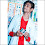## Introduction:

Dynamic programming is a powerful technique used to solve complex problems by breaking them down into smaller, overlapping subproblems. One such problem that can be efficiently solved using dynamic programming is the edit distance problem. In this article, we will explore the concept of edit distance and provide an implementation using a tabular approach.

## Problem Statement:

The edit distance between two strings is the minimum number of operations required to transform one string into another. The allowed operations are:

1. Insertion: Add a character to the string.
2. Deletion: Remove a character from the string.
3. Substitution: Replace a character with another.

For example, consider two strings "sarthak" and "deepak." The edit distance between them is 5 since we need 5 operations to transform "sarthak" into "deepak" (s -> d, a -> e, r -> e, t -> p, h -> a).

## Code:

#include<iostream>
#include<string.h>
using namespace std;

int editDistance(string s1,string s2,int m,int n){

int dp[m+1][n+1];

for(int i=0;i<=m;i++)
dp[i]=i;

for(int j=0;j<=n;j++)
dp[j]=j;

for(int i=1;i<=m;i++){

for(int j=1;j<=n;j++){

if(s1[i-1]==s2[j-1]){

dp[i][j]=dp[i-1][j-1];
}
else
{
dp[i][j]=1+min(dp[i-1][j],(dp[i][j-1],dp[i-1][j-1]));
}

}

}
return dp[m][n];
}
int main(){

string s1="sarthak",s2="deepak";

int m=7,n=6;

cout<<editDistance(s1,s2,m,n);
}

6

## Explaination

Tabular Approach Implementation: To solve the edit distance problem using dynamic programming, we can utilize a tabular approach. Let's understand the implementation step by step:

1. Define the function:

• The function takes two strings, s1 and s2, along with their respective lengths, m and n.
• It returns the minimum edit distance between s1 and s2.
2. Initialize the dynamic programming table:

• Create a 2D array, dp, of size (m+1) x (n+1), representing the dynamic programming table.
• dp[i][j] will store the edit distance between the substrings s1[0...i-1] and s2[0...j-1].
3. Initialize the base cases:

• For i = 0 to m, dp[i] is initialized as i, representing the number of deletions required to convert s1[0...i-1] to an empty string.
• For j = 0 to n, dp[j] is initialized as j, representing the number of insertions required to convert an empty string to s2[0...j-1].
4. Fill in the table:

• For i = 1 to m and j = 1 to n:
• If s1[i-1] is equal to s2[j-1], then no operation is required. Hence, dp[i][j] = dp[i-1][j-1].
• Otherwise, we need to choose the minimum of the following three options:
• dp[i-1][j] + 1: Deletion operation. Move from s1[0...i-2] to s2[0...j-1].
• dp[i][j-1] + 1: Insertion operation. Move from s1[0...i-1] to s2[0...j-2].
• dp[i-1][j-1] + 1: Substitution operation. Move from s1[0...i-2] to s2[0...j-2].
5. Return the result:

• After filling in the table, the minimum edit distance between s1 and s2 will be stored in dp[m][n]. Return this value.

## Conclusion:

The edit distance problem is a classic example of how dynamic programming can provide an efficient solution. By utilizing a tabular approach, we can break down the problem into smaller subproblems and store their results in a table. This allows us to avoid redundant calculations and solve the problem optimally. Understanding and implementing concepts like edit distance using dynamic programming can greatly enhance our problem-solving skills.

1.